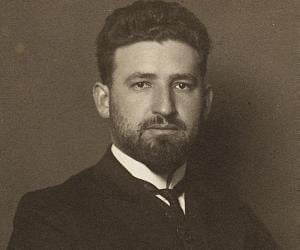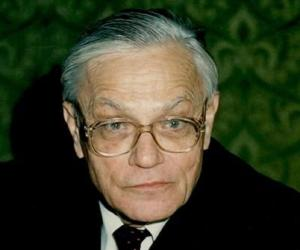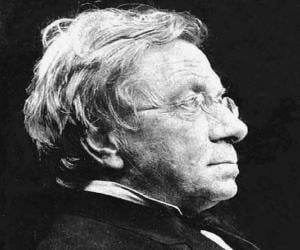Swiss mathematicians have casted an overpowering effect on the field of mathematics. They are lauded for their ground-breaking contribution in the field of calculus, trigonometry, geometry and so on. Their research and findings led to the discovery of many unknown facts and facets of mathematics which helped in the better understanding of the subject. While talking of Swiss mathematicians, the first name that strikes the mind is that of Jacob Bernoulli. He has through his research delivered to the world the first type of law of large numbers in the probability theory. He has also contributed greatly to the parallels of logic, algebra and geometry. His brother, Johann Bernoulli, is credited for developing the isochrone and tautchrone concepts and made major contributions to differential equations, optics, clock construction and mathematics of ship sails. Leonhard Euler, the famous Swiss mathematician, introduced mathematical notations and terminology such as the concepts of mathematical functions. In the 20th century Switzerland, Paul Bernays played a pivotal role in expanding the knowledge of mathematics further. He developed the finitist concept and made vast contribution by developing the axiomatic set theory and the philosophy of mathematics. Check this section further to find out more about Swiss mathematician, their life, profile, works and timeline.Marcel Grossmann
09 April 1878
MathematicianNicolas Fatio de Duillier
16 February 1664
astronomer, mathematicianGabriel Cramer
31 July 1704
Mathematician, Physicist, University teacherJakob Steiner
18 March 1796
MathematicianArmand Borel
21 May 1923
Swiss mathematicianJohann Jakob Balmer
01 May 1825
Swiss mathematicianJakob Bernoulli
01 January 1655
MathematicianJohann Balmer
01 May 1825Rudolf Wolf
07 July 1816
AstronomerJoost Bürgi
28 February 1552
Mathematician